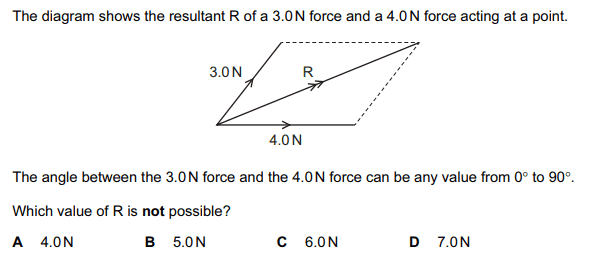# Resultant Vector

According to my understanding, option D is the only possible value of R. I don't understand how options A, B and C are included. Please explain this question.
Thanks.
(regards)## Answers and Replies

drvrm
According to my understanding, option D is the only possible value of R. I don't understand how options A, B and C are included. Please explain this question.
Thanks.
(regards)

just try to check the values of resultant at 0 and 90 degrees
anything outside this range of R is not possible.

just try to check the values of resultant at 0 and 90 degrees
anything outside this range of R is not possible.
I do not understand...

drvrm
I do not understand...

in the question the angles are given between zero and ninety degree
so if you put zero
then the two vectors are collinear so they will add up that gives you a value of R
i think that is maximum value
similarly put them at 90 degree

in the question the angles are given between zero and ninety degree
so if you put zero
then the two vectors are collinear so they will add up that gives you a value of R
i think that is maximum value
similarly put them at 90 degree
If we put them at 90 degrees, then the vectors will still add up to 7 N, won't they?

Mentor
If we put them at 90 degrees, then the vectors will still add up to 7 N, won't they?
Are you familiar with how to do vector addition?

drvrm
If we put them at 90 degrees, then the vectors will still add up to 7 N, won't they?

two vectors at an angle theta then there is a rule parallelogram -law
R = sqrt{ 3^2 + 4^2 + 2 (3*4 ) cos (theta) }
so if theta =90
cos 90 =0

Are you familiar with how to do vector addition?
Yes..

Mentor
Yes..
Then you know that, unless they are co-linear and pointing in the same direction, their resultant magnitude can't be 7.

•two vectors at an angle theta then there is a rule parallelogram -law
R = sqrt{ 3^2 + 4^2 + 2 (3*4 ) cos (theta) }
so if theta =90
cos 90 =0
Oh I understand now, it means that if we put Cos 90, then the answer will be the lowest value. Similarly if we put Cos 0, then the outcome will be the maximum value. Maximum value=7 Minimum Value=5 so 4N is not possible. Am I correct?

Then you know that, unless they are co-linear and pointing in the same direction, their resultant magnitude can't be 7.
When they will be co-linear, the resultant magnitude will be 7N, and when they are perpendicular to each other then the resultant magnitude will be 5N, so the value of R must lie between 5-7N. In the above MCQ the answer will be A.4N because it is not a possible value of R. Am I right?

Mentor
When they will be co-linear, the resultant magnitude will be 7N, and when they are perpendicular to each other then the resultant magnitude will be 5N, so the value of R must lie between 5-7N. In the above MCQ the answer will be A.4N because it is not a possible value of R. Am I right?
Yes.

Yes.
Thanks Sir.

two vectors at an angle theta then there is a rule parallelogram -law
R = sqrt{ 3^2 + 4^2 + 2 (3*4 ) cos (theta) }
so if theta =90
cos 90 =0
Thanks a lot! I understand now!!

•drvrm